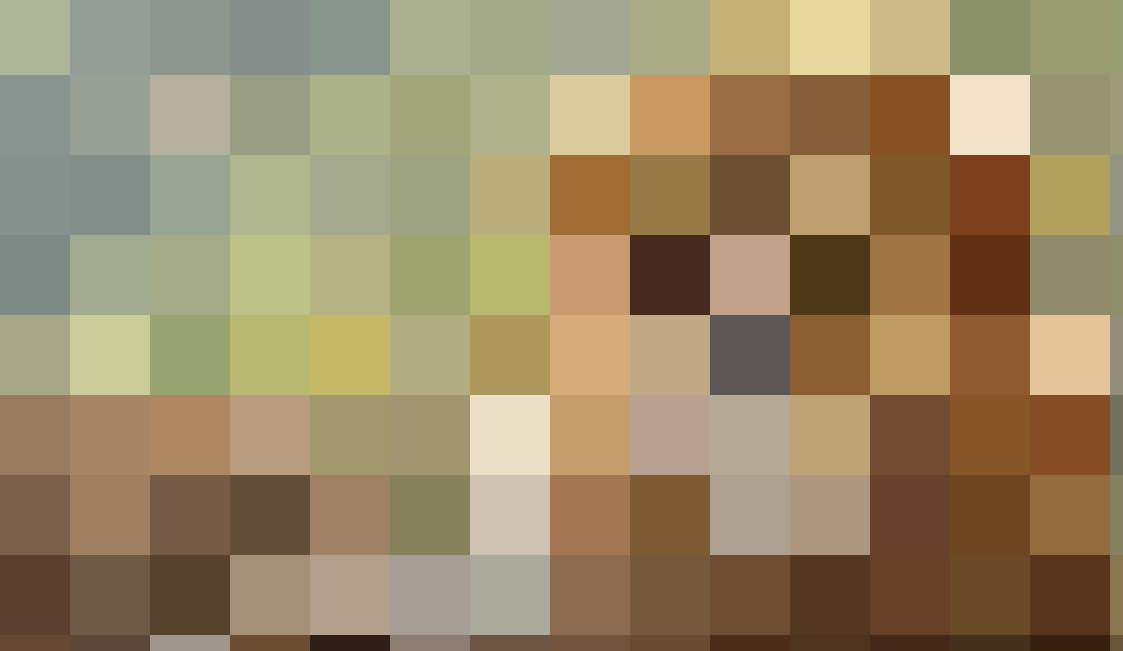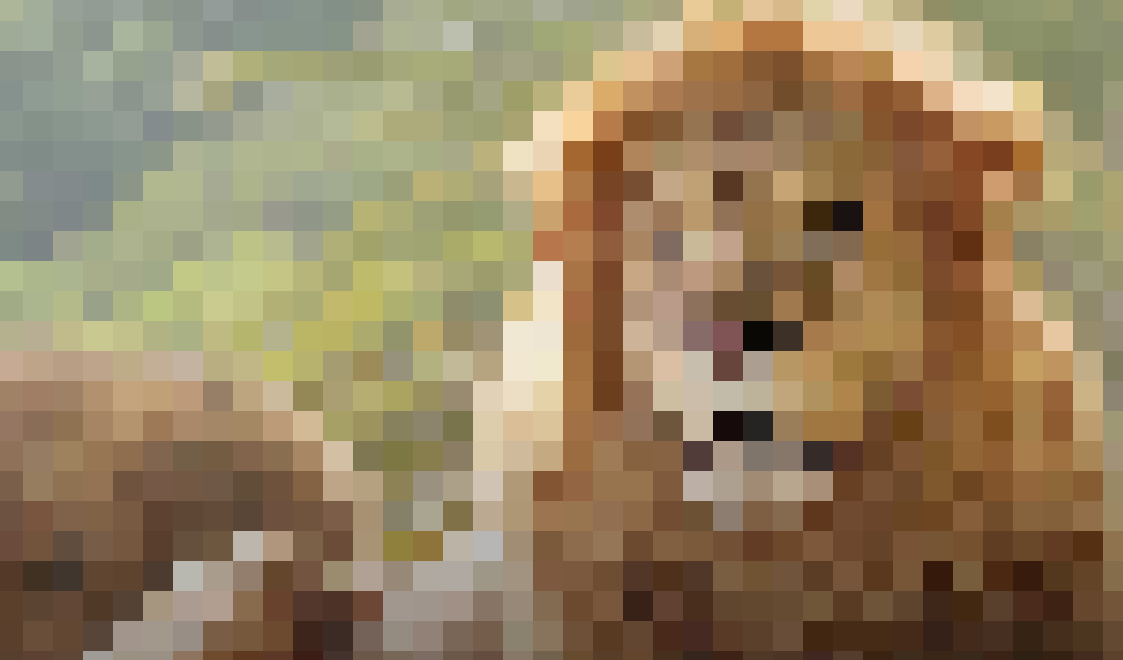# [JAVA] Maybe it can be used at a year-end party? Mosaic game code

### Background and purpose

As the year-end party season approaches, the stress of newcomers who are forced to be secretaries in the middle is considerable. Maybe it can be used as a sideshow for a year-end party to reach out to such a newcomer. I created a mosaic game with Processing.

### how to use

Click `k` on the keyboard to reduce the image mosaic Click `j` on your keyboard to enlarge the image mosaic.

``````PImage img;
MozImage moz;
float mozSize = 80;//Early mosaic size
boolean sizeUp = true;
boolean sizeDown = true;

void setup() {
background(0);
size(1143, 676);//Set canvas size to image size

smooth();
noStroke();
}

void draw() {
for (int i=0; i<width/mozSize; i++) {
float x = mozSize * i;
for (int j=0; j<height/mozSize; j++) {
float y = mozSize * j;
moz = new MozImage(int(x), int(y));
moz.show();
}
}
}

void keyPressed() {
if (sizeDown == true && key == 'k') {
if(mozSize >10){
mozSize -= 10;
}else{
mozSize = 1;
}
sizeDown = false;
} else if (sizeUp == true && key == 'j') {
mozSize += 10;
sizeUp = false;
}
}

void keyReleased() {
sizeDown = true;
sizeUp = true;
}

class MozImage {
float x;
float y;
float r;
MozImage(int _x, int _y) {
x = _x;
y = _y;
r = mozSize;
}
void show() {
color c = img.get(int(x), int(y));
fill(c);
noStroke();
//ellipse(x,y,2*r,2*r);
rect(x, y, r, r);
}
}

``````

### Example of use:

Problem: What is this animal!

Image at the start of the gameGradually reduce the mosaic ...